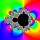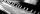Geometric mean

Calculate the geometric mean of numbers a=15.2 and b=25.6. Determine the mean by construction where a and b are the length of the lines.

Result

m =  19.726

Solution:Leave us a comment of example and its solution (i.e. if it is still somewhat unclear...):Be the first to comment!To solve this example are needed these knowledge from mathematics:

Looking for a statistical calculator?

Next similar examples:

1. Geometric progression 2There is geometric sequence with a1=5.7 and quotient q=-2.5. Calculate a17.
2. Tenth memberCalculate the tenth member of geometric sequence when given: a1=1/2 and q=2
3. Geometric progressionFill 4 numbers between 4 and -12500 to form geometric progression.
4. CoefficientDetermine the coefficient of this sequence: 7.2; 2.4; 0.8
5. GP - 8 itemsDetermine the first eight members of a geometric progression if a9=512, q=2
6. Five membersWrite first 5 members geometric sequence and determine whether it is increasing or decreasing: a1 = 3 q = -2
7. Six termsFind the first six terms of the sequence a1 = -3, an = 2 * an-1
8. Geometric progression 48,4√2,4,2√2
9. PianoIf Suzan practicing 10 minutes at Monday; every other day she wants to practice 2 times as much as the previous day, how many hours and minutes will have to practice on Friday?
10. ProductThe product of two consecutive odd numbers is 8463. What are this numbers?
11. PIN - codesHow many five-digit PIN - code can we create using the even numbers?
12. CalculationHow much is sum of square root of six and the square root of 225?
13. Theorem proveWe want to prove the sentence: If the natural number n is divisible by six, then n is divisible by three. From what assumption we started?
14. PowerNumber ?. Find the value of x.
15. ExaminationThe class is 21 students. How many ways can choose two to examination?Solve quadratic equation: 2x2-58x+396=0Solve the system of linear equations with three unknowns: A + B + C = 14 B - A - C = 4 2A - B + C = 0